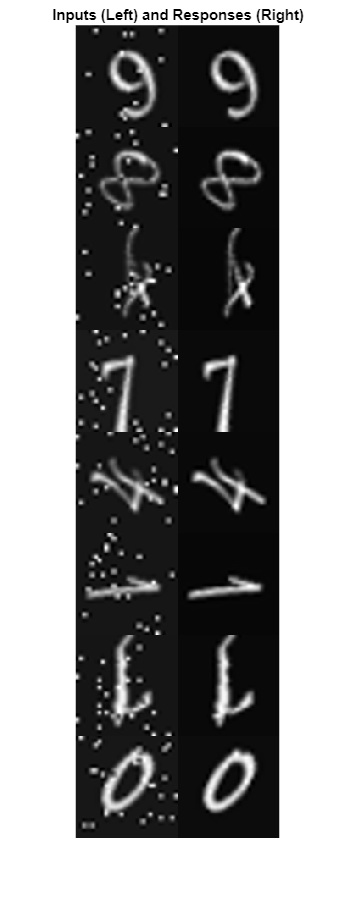# 为图像到图像的回归准备数据存储

### 使用预处理管道准备数据

```digitDatasetPath = fullfile(matlabroot,"toolbox","nnet", ... "nndemos","nndatasets","DigitDataset"); imds = imageDatastore(digitDatasetPath, ... IncludeSubfolders=true,LabelSource="foldernames");```

`imds.ReadSize = 500;`

`imds = shuffle(imds);`

`[imdsTrain,imdsVal,imdsTest] = splitEachLabel(imds,0.95,0.025);`

```dsTrainNoisy = transform(imdsTrain,@addNoise); dsValNoisy = transform(imdsVal,@addNoise); dsTestNoisy = transform(imdsTest,@addNoise);```

```dsTrain = combine(dsTrainNoisy,imdsTrain); dsVal = combine(dsValNoisy,imdsVal); dsTest = combine(dsTestNoisy,imdsTest);```

```dsTrain = transform(dsTrain,@commonPreprocessing); dsVal = transform(dsVal,@commonPreprocessing); dsTest = transform(dsTest,@commonPreprocessing);```

`dsTrain = transform(dsTrain,@augmentImages);`

### 预览经过预处理的数据

```exampleData = preview(dsTrain); inputs = exampleData(:,1); responses = exampleData(:,2); minibatch = cat(2,inputs,responses); montage(minibatch',Size=[8 2]) title("Inputs (Left) and Responses (Right)")```### 定义卷积自编码器网络

`imageLayer = imageInputLayer([32,32,1]);`

```encodingLayers = [ ... convolution2dLayer(3,8,Padding="same"), ... reluLayer, ... maxPooling2dLayer(2,Padding="same",Stride=2), ... convolution2dLayer(3,16,Padding="same"), ... reluLayer, ... maxPooling2dLayer(2,Padding="same",Stride=2), ... convolution2dLayer(3,32,Padding="same"), ... reluLayer, ... maxPooling2dLayer(2,Padding="same",Stride=2)];```

```decodingLayers = [ ... transposedConv2dLayer(2,32,Stride=2), ... reluLayer, ... transposedConv2dLayer(2,16,Stride=2), ... reluLayer, ... transposedConv2dLayer(2,8,Stride=2), ... reluLayer, ... convolution2dLayer(1,1,Padding="same"), ... clippedReluLayer(1.0), ... regressionLayer]; ```

`layers = [imageLayer,encodingLayers,decodingLayers];`

### 定义训练选项

```options = trainingOptions("adam", ... MaxEpochs=50, ... MiniBatchSize=imds.ReadSize, ... ValidationData=dsVal, ... ValidationPatience=5, ... Plots="training-progress", ... OutputNetwork="best-validation-loss", ... Verbose=false);```

### 训练网络

```net = trainNetwork(dsTrain,layers,options); modelDateTime = string(datetime("now",Format="yyyy-MM-dd-HH-mm-ss")); save("trainedImageToImageRegressionNet-"+modelDateTime+".mat","net"); ```

### 评估去噪网络的性能

`ypred = predict(net,dsTest);`

`testBatch = preview(dsTest);`

```idx = 1; y = ypred(:,:,:,idx); x = testBatch{idx,1}; ref = testBatch{idx,2}; montage({x,y})````psnrNoisy = psnr(x,ref)`
```psnrNoisy = single 19.6457 ```
`psnrDenoised = psnr(y,ref)`
```psnrDenoised = single 20.6994 ```

### 支持函数

`addNoise` 辅助函数使用 `imnoise` (Image Processing Toolbox) 函数在图像中添加椒盐噪声。`addNoise` 函数要求输入数据的格式为图像数据元胞数组，这与 `ImageDatastore``read` 函数返回的数据格式相匹配。

```function dataOut = addNoise(data) dataOut = data; for idx = 1:size(data,1) dataOut{idx} = imnoise(data{idx},"salt & pepper"); end end```

`commonPreprocessing` 辅助函数定义训练集、验证集和测试集共有的预处理。该辅助函数执行以下预处理步骤。

1. 将图像数据转换为数据类型 `single`

2. 使用 `imresize` 函数调整图像数据的大小以匹配输入层的大小。

3. 使用 `rescale` 函数将数据归一化到范围 [0, 1]。

```function dataOut = commonPreprocessing(data) dataOut = cell(size(data)); for col = 1:size(data,2) for idx = 1:size(data,1) temp = single(data{idx,col}); temp = imresize(temp,[32,32]); temp = rescale(temp); dataOut{idx,col} = temp; end end end```

`augmentImages` 辅助函数使用 `rot90` 函数在数据中添加随机化的 90 度旋转值。相同的旋转应用于网络输入和对应的预期响应。函数要求输入数据的格式为图像数据的两列元胞数组，这与 `CombinedDatastore``read` 函数返回的数据格式相匹配。

```function dataOut = augmentImages(data) dataOut = cell(size(data)); for idx = 1:size(data,1) rot90Val = randi(4,1,1)-1; dataOut(idx,:) = {rot90(data{idx,1},rot90Val), ... rot90(data{idx,2},rot90Val)}; end end```